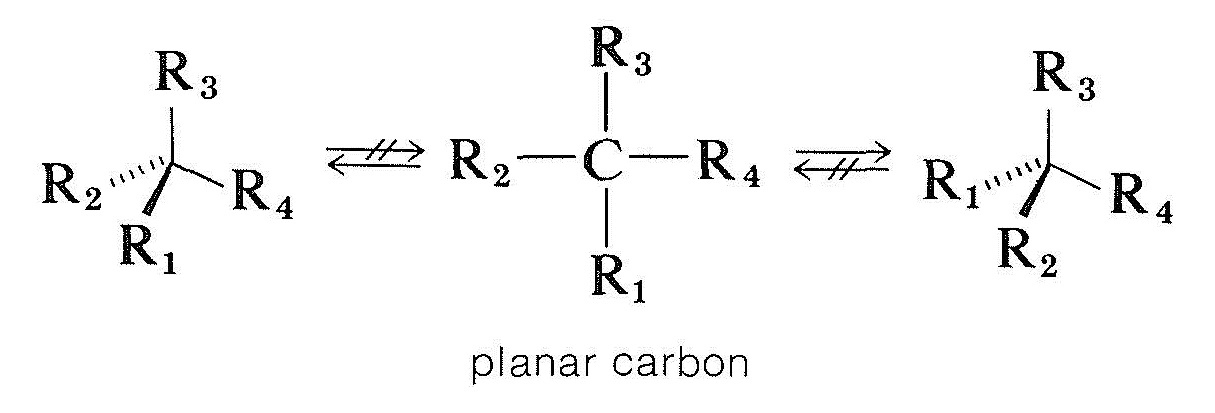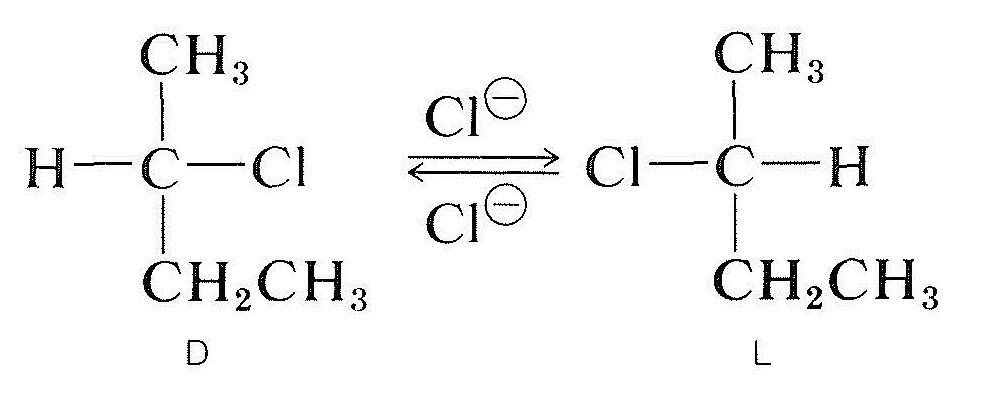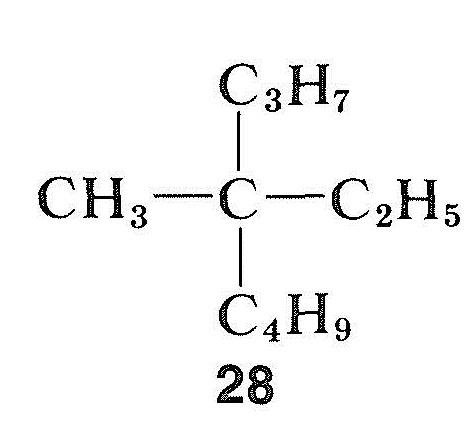# 19.11: Racemization

$$\newcommand{\vecs}{\overset { \rightharpoonup} {\mathbf{#1}} }$$ $$\newcommand{\vecd}{\overset{-\!-\!\rightharpoonup}{\vphantom{a}\smash {#1}}}$$$$\newcommand{\id}{\mathrm{id}}$$ $$\newcommand{\Span}{\mathrm{span}}$$ $$\newcommand{\kernel}{\mathrm{null}\,}$$ $$\newcommand{\range}{\mathrm{range}\,}$$ $$\newcommand{\RealPart}{\mathrm{Re}}$$ $$\newcommand{\ImaginaryPart}{\mathrm{Im}}$$ $$\newcommand{\Argument}{\mathrm{Arg}}$$ $$\newcommand{\norm}{\| #1 \|}$$ $$\newcommand{\inner}{\langle #1, #2 \rangle}$$ $$\newcommand{\Span}{\mathrm{span}}$$ $$\newcommand{\id}{\mathrm{id}}$$ $$\newcommand{\Span}{\mathrm{span}}$$ $$\newcommand{\kernel}{\mathrm{null}\,}$$ $$\newcommand{\range}{\mathrm{range}\,}$$ $$\newcommand{\RealPart}{\mathrm{Re}}$$ $$\newcommand{\ImaginaryPart}{\mathrm{Im}}$$ $$\newcommand{\Argument}{\mathrm{Arg}}$$ $$\newcommand{\norm}{\| #1 \|}$$ $$\newcommand{\inner}{\langle #1, #2 \rangle}$$ $$\newcommand{\Span}{\mathrm{span}}$$$$\newcommand{\AA}{\unicode[.8,0]{x212B}}$$

Optically active biphenyl derivatives (Section 13-5A) are racemized if the two aromatic rings at any time pass through a coplanar configuration by rotation about the central bond. This can be brought about more or less easily by heat, unless the 2,2'-ortho substituents are very large.

The way in which compounds with asymmetric carbon atoms are racemized is more complicated. One possibility would be for a tetrahedral chiral carbon attached to four groups to become planar and achiral without breaking any bonds. Theoretical calculations indicate that this is not a likely process for chiral tetravalent carbon but, as we will see, it does occur with chiral carbon and other chiral atoms that are attached to three groups:Optically active carbonyl compounds of the type $$\ce{-CHC=O}$$, in which the alpha carbon is asymmetric, are racemized by both acids and bases, and from Section 17-1 we can be sure that this is related to enolization. Formation of either the enol or the enolate anion will destroy the asymmetry of the $$\alpha$$ carbon so that, even if only trace amounts of enol are present at any given time, eventually all of the compound will be racemized. However, the mechanism requires both that there be an $$\alpha$$ hydrogen and that the center of symmetry be located at this $$\alpha$$ carbon. Otherwise, acids and bases are ineffective in catalyzing racemization.The racemization of an optically active secondary halide with the chiral carbon carrying the halogen (e.g., 2-chlorobutane) may occur ih solution and, usually, the more polar and better ionizing the solvent is, the more readily the substance is racemized. Ionization of the halide by an $$S_\text{N}1$$ process probably is responsible, and this certainly would be promoted by polar solvents (Section 8-6). All indications are that an alkyl carbocation once dissociated from its accompanying anion is planar; and, when such an ion recombines with the anion, it has equal probability of forming the $$D$$ and $$L$$ enantiomers:Optically active halides also can be racemized by an $$S_\text{N}2$$ mechanism. A solution of active 2-chlorobutane in 2-propanone containing dissolved lithium chloride becomes racemic. Displacement of the chloride of the halide by chloride ion inverts configuration at the atom undergoing substitution (see Section 8-5). A second substitution regenerates the original enantiomer. Eventually, this back-and-forth process produces equal numbers of the $$D$$ and $$L$$ forms; the substance then is racemic:Asymmetric alcohols often are racemized by strong acids. Undoubtedly, ionization takes place, and recombination of the carbocation with water leads to either enantiomer:In contrast to halides, alcohols, and carbonyl compounds, hydrocarbons may be extremely difficult to racemize. This is particularly true for a compound with a quaternary asymmetric center, such as methylethylpropylbutylmethane, $$28$$, which has no "handle" to allow one to convert the asymmetric carbon to a symmetric condition by simple chemical means:However, hydrocarbons that have a hydrogen atom at the asymmetric carbon may be racemized if they can be converted either to carbocations or to carbanions. The ease of carbanion-type racemization will depend on the acidity of the attached hydrogen and on the stereochemical stability of the intermediate carbanion that is formed. If the configuration of the carbanion intermediate inverts, racemization will result (also see Section 6-4E):The carbocation type of racemization of an optically active hydrocarbon can occur by the exchange reaction described in Section 10-9.